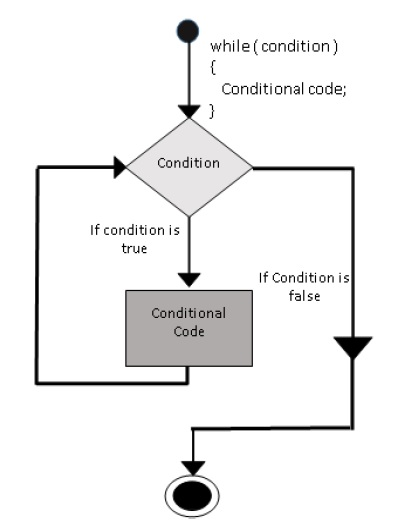# Dart Programming - while Loop

The while loop executes the instructions each time the condition specified evaluates to true. In other words, the loop evaluates the condition before the block of code is executed.

The following illustration shows the flowchart of the while loop −Following is the syntax for the while loop.

```while (expression) {
Statement(s) to be executed if expression is true
}
```

## Example

```void main() {
var num = 5;
var factorial = 1;

while(num >=1) {
factorial = factorial * num;
num--;
}
print("The factorial  is \${factorial}");
}
```

The above code uses a while loop to calculate the factorial of the value in the variable num.

The following output is displayed on successful execution of the code.

```The factorial is 120
```
dart_programming_loops.htm